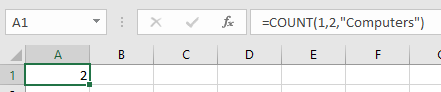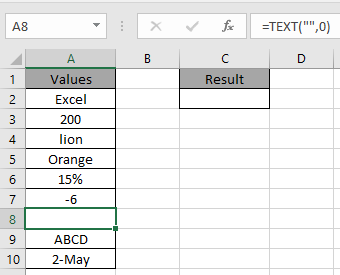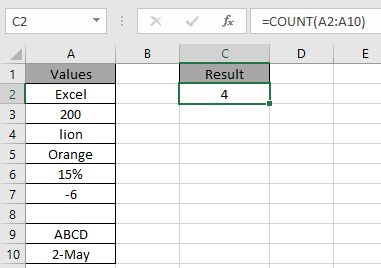# How to Use COUNT Function in Excel

COUNT function is a built in Excel function to calculate the Count of only numeric values in the range.
Syntax:

=COUNT (value1, [value2], ...)
Note:
Empty cells and text are not considered by COUNT function

If we write a formula

=COUNT(1,2,” Computers”)

It will return 2 as the count of numeric values which are 1 & 2.Let’s understand more of this function using it an example.Here we have some values in column A including the formula in A8 cell.
Use the formula to count cells having only numeric values. It includes any number, date and time.

<div="bbbb">=COUNT(A2:A10)4 is the count of numeric value cells which are A3, A6, A7 and A10.
Use the COUNTA function to count text and logic_values in Excel.
Hope you understood how to use COUNTA function in Excel. Explore more articles on built-in Excel function here. Please feel free to state your query or feedback for the above article.

Popular Articles:

50 Excel Shortcuts to Increase Your Productivity

How to use the VLOOKUP Function in Excel

How to use the COUNTIF function in Excel 2016

How to Use SUMIF Function in Excel

Terms and Conditions of use

The applications/code on this site are distributed as is and without warranties or liability. In no event shall the owner of the copyrights, or the authors of the applications/code be liable for any loss of profit, any problems or any damage resulting from the use or evaluation of the applications/code.